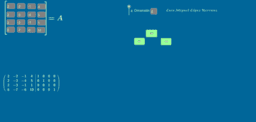Matrices

Parent topic:
Algebra
•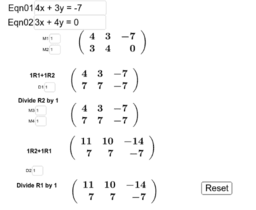Gauss-Jordan 2x2 Elimination

Activity

A B Cron

•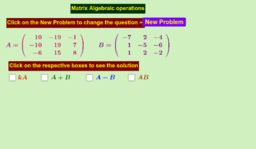Matrices Basic operations

Activity

D.Vasu Raj

•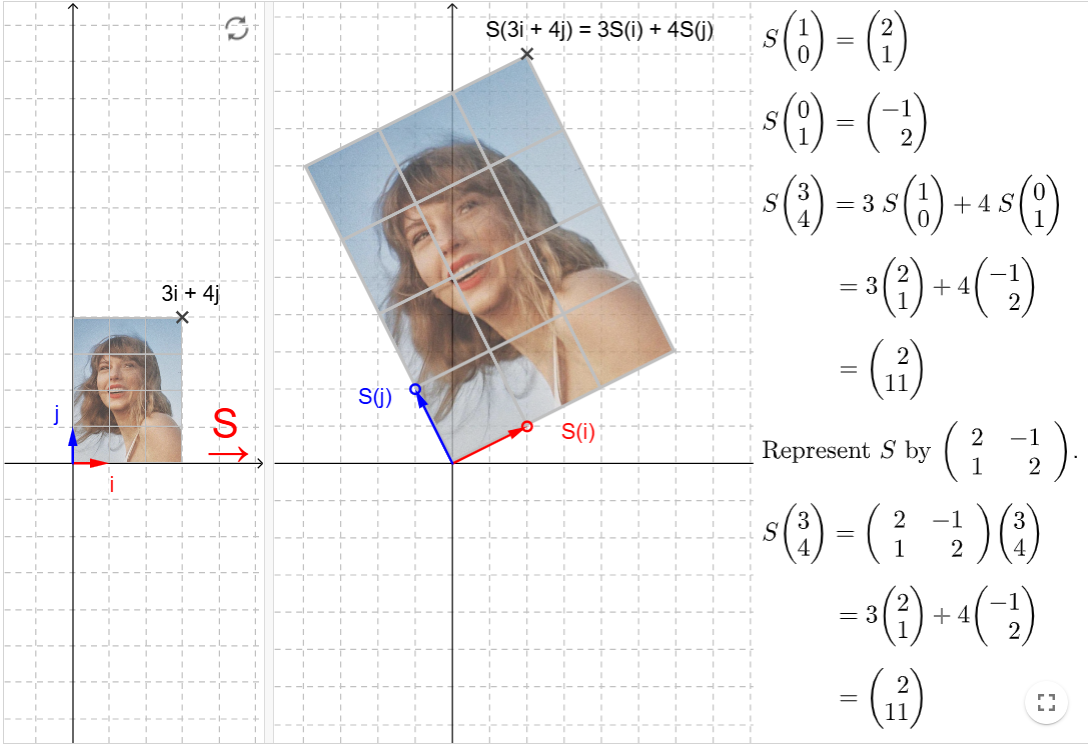Matrix and Linear Transformation (HTML5 version)

Activity

Anthony OR 柯志明

•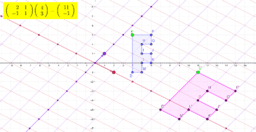Images of F: Matrix Transformations

Activity

Steve Phelps

•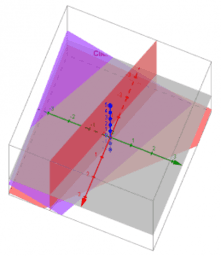Linear Algebra

Book

Kevin Hopkins

•Applications of Matrices and Determinants

Activity

D.Vasu Raj

•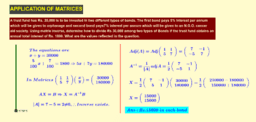Application of matrices-problem

Activity

D.Vasu Raj

•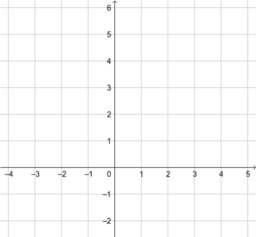Entering Matrices

Activity

GeoGebra Institute of MEI

•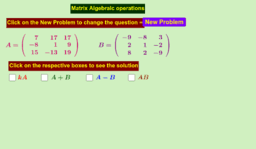Matrices - Algebraic operations

Activity

D.Vasu Raj

•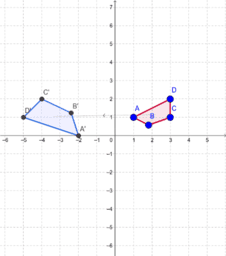Transformation matrices

Activity

Jon Ingram

•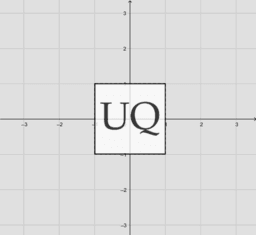Geometric properties of matrices

Activity

Juan Carlos Ponce Campuzano

•矩陣與線性變換

Activity

Anthony OR 柯志明

•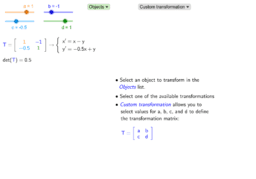Lesson & Practice: Matrices and Linear Transformations

Activity

Simona Riva

•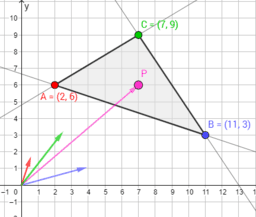matrices in CAS

Activity

chris cambré

•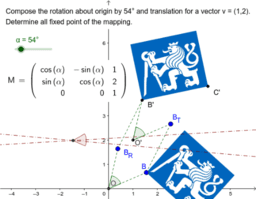Affine transformation

Activity

Šárka Voráčová

•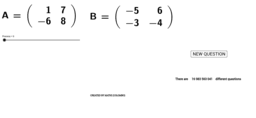Multiplying two matrices

Activity

Mathiyalagan

•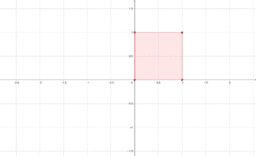Matrix Transformations

Activity

Emma

•Linear transformations

Activity

•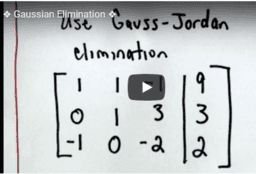Gaussian method of elimination

Activity

D.Vasu Raj

•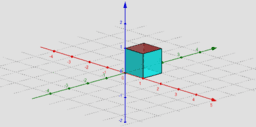Activity 4: Matrices as linear transformations

Activity

Juan Carlos Ponce Campuzano

•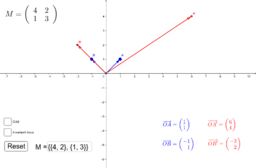Eigenvalues & Eigenvectors

Activity

Lee Robinson

•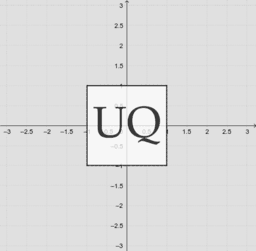A geometric interpretation of matrices

Book

Juan Carlos Ponce Campuzano

•Matrices: Unit PLan

Book

•